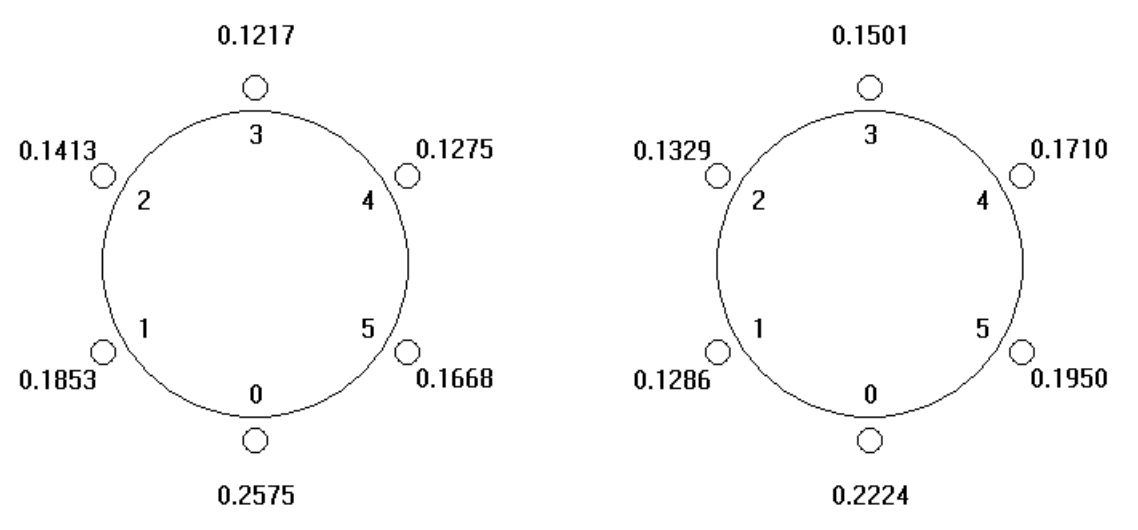시간 제한 메모리 제한 제출 정답 맞은 사람 정답 비율
2 초 512 MB 5 3 2 66.667%

문제

The game of Left-Right-Win is played by n players around a table with a spinner* which returns Left with probability P_left, Right with probability P_right and Win with probability 1 – P_left – P_right.

The game begins with the starting player (labeled 0 in the figures with other players labeled 1 .. (n-1) clockwise) who spins the spinner. If the result is Win, he wins the pot of say \$100. If the result is Left, the spinner is passed to the player on his/her left (player 1). If the result is Right, the spinner is passed to the player on his/her right (player (n-1)). The new holder of the spinner repeats this process: winning or passing the spinner to the left or right. The game continues until someone wins. (Since the game could, theoretically, go on forever, there is a stopping rule such as: after 2n turns, the house takes the pot.) The figure on left shows the probabilities of each of 6 players winning with P_left = 0.5 and P_right = 0.4. The figure on the right shows the probabilities of each of 6 players winning with P_left = 0.1 and P_right = 0.8.Clearly, player 3 is not going to want to pay as much as player 0 to play the game. We would like each player to contribute to the pot an amount proportional to the probabilyt of their winning it (the potentially infinite game). Write a program which computes the amount each player should contribute to a \$100 pot given P_left and P_right.

Computations should be done in double precision floating point.

* A spinner is a device which returns one of several values with specified probabilities for each value. Originally, a spinner was a balanced pointer on a card with arcs around the center labeled with the different outcomes. You spin the pointer and where it lands is the chosen value. The arc lengths are proportional to the probabilities of the values. A spinner may be simulated by any random device. For instance, the P_left = 1/3 and P_right = 1/3 may be simulated by rolling a single die and specifying Left for 1 or 2, Right for 3 or 4 and Win for 5 or 6.

입력

The first line of input contains a single decimal integer P, (1 ≤ P ≤ 100), which is the number of data sets that follow. Each data set should be processed identically and independently.

Each data set consists of a single line of input containing 5 space separated values: the data set number, K, followed by the number of players, n, a decimal integer: (3 ≤ n ≤ 15), followed by the label of the player k, a decimal integer, (0 ≤ k ≤ n-1), whose contribution is to be found, followed by two floating point values P_left and P_right. (P_left+P_right ≥ 0.8).

3
1 6 0 .5 .4
2 6 3 .1 .8
3 6 2 .9 .03

1 25.75
2 15.01
3 17.13